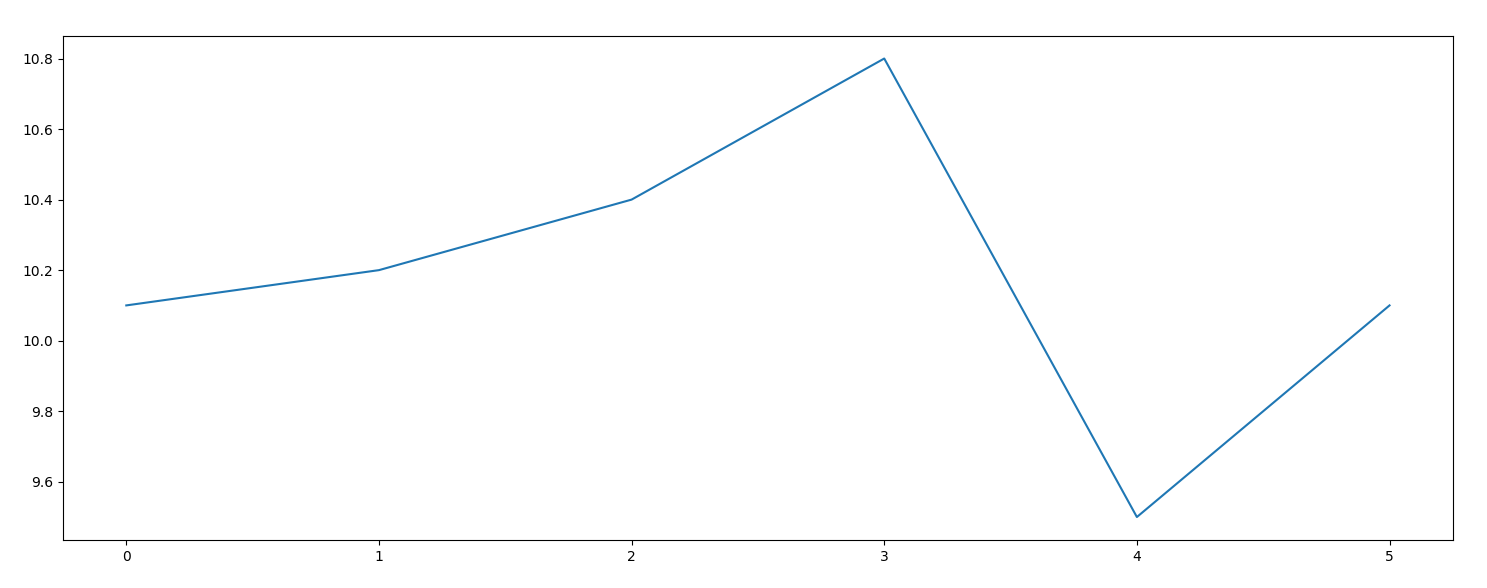# Funliner: Handy plot tool

## 1 Introduction

It's very command to need to parse a log file for numbers and then plot that data. Wouldn't it be nice to have a simple tool you could pipe numbers to for quick visualization?

## 2 Plot(.py)

Here is the code for our python program:

```#!/usr/bin/env python3

import matplotlib.pyplot as plt
import sys

plt.plot([float(x) for x in sys.stdin],sys.argv if len(sys.argv)>1 else '-')

plt.show()
```

You should `chmod +x plot` after saving this file.

## 3 How to use it

Now suppose you had the following log file:

```current error: 10.1 continuing
current error: 10.2 continuing
current error: 10.4 continuing
current error: 10.8 continuing
current error: 9.5 continuing
current error: 10.1 continuing
```

Let's assume it is saved as example.txt You could visualize this as follows:

```\$ sed -e 's/[^0-9.]*//g' example.txt  | ./plot
```

And you'd get this output:One more nice feature is that we can customize the plot style by passing arguments to plot: Try it out for yourself:

```\$ sed -e 's/[^0-9.]*//g' example.txt  | ./plot o
```

Created: 2020-08-19 Wed 16:01

Emacs 25.1.1 (Org mode 8.2.10)

Validate